81888160 81888665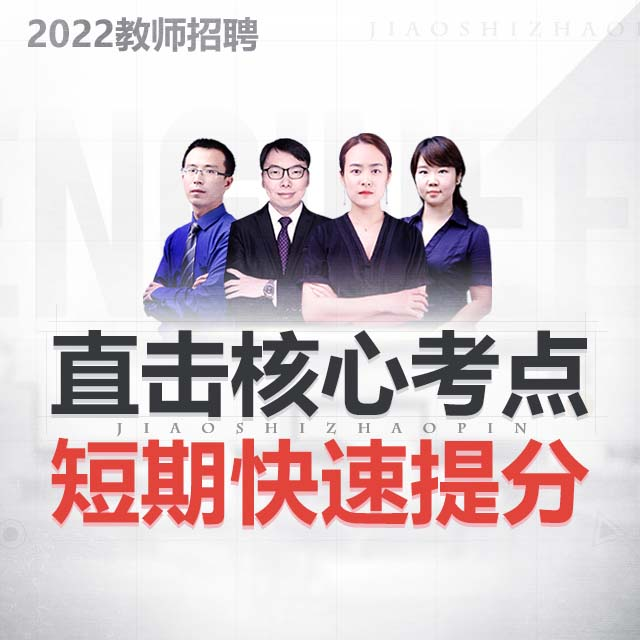### 2022教师招聘 笔试套餐班

¥

• 新用户注册,即送6张优惠券,购课即可抵用
• 累计支付满300,再次购课享8.5折,新老优惠不同享

• 北京
• 天津
• 上海
• 江苏
• 浙江
• 山东
• 江西
• 安徽
• 广东
• 广西
• 海南
• 辽宁
• 吉林
• 黑龙江
• 内蒙古
• 山西
• 福建
• 河南
• 河北
• 湖南
• 湖北
• 四川
• 重庆
• 云南
• 贵州
• 新疆
• 西藏
• 陕西
• 青海
• 宁夏
• 甘肃
• 全国

（课时共 h）

• 可免费重学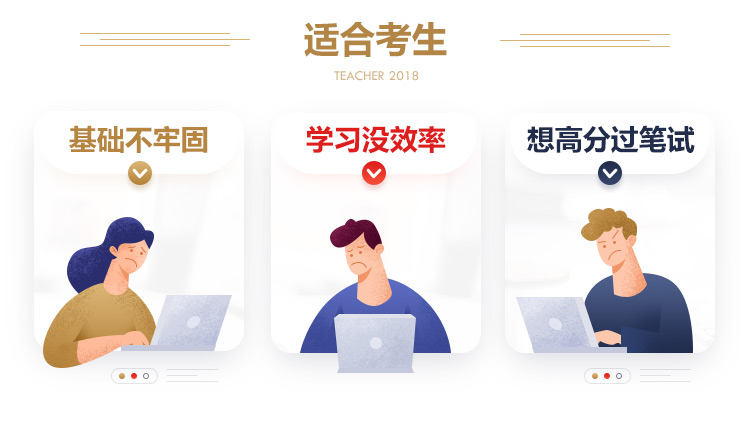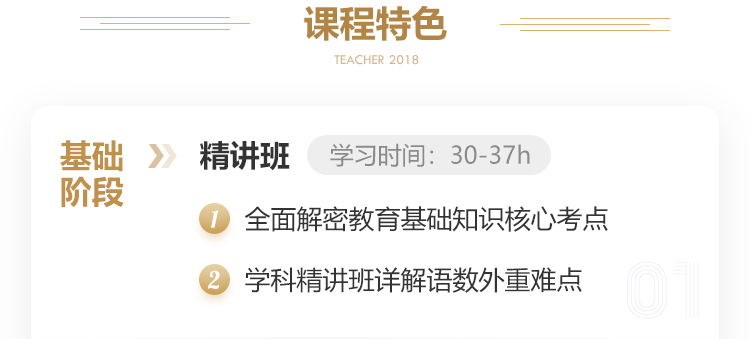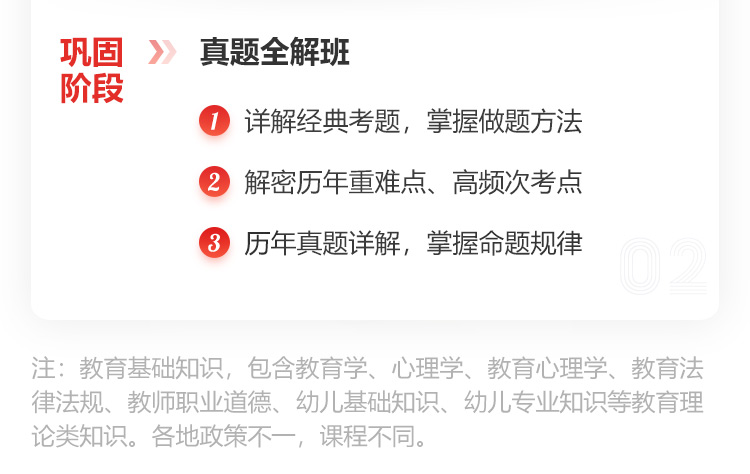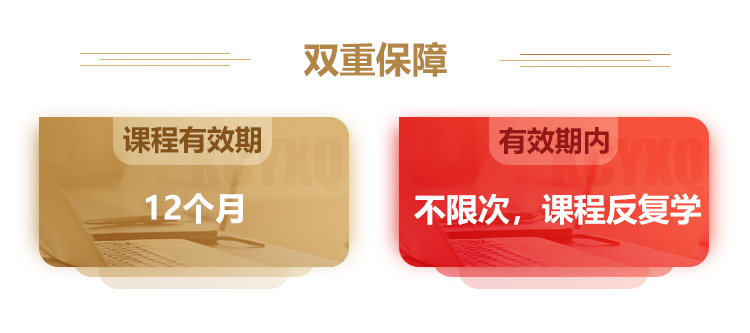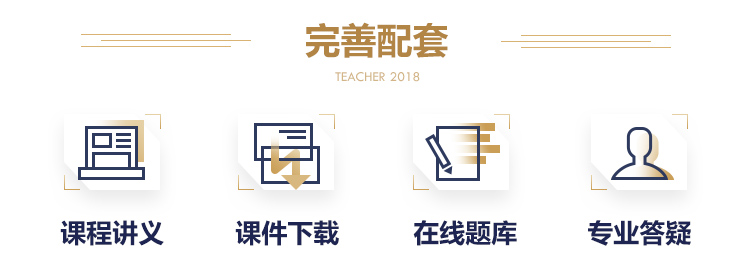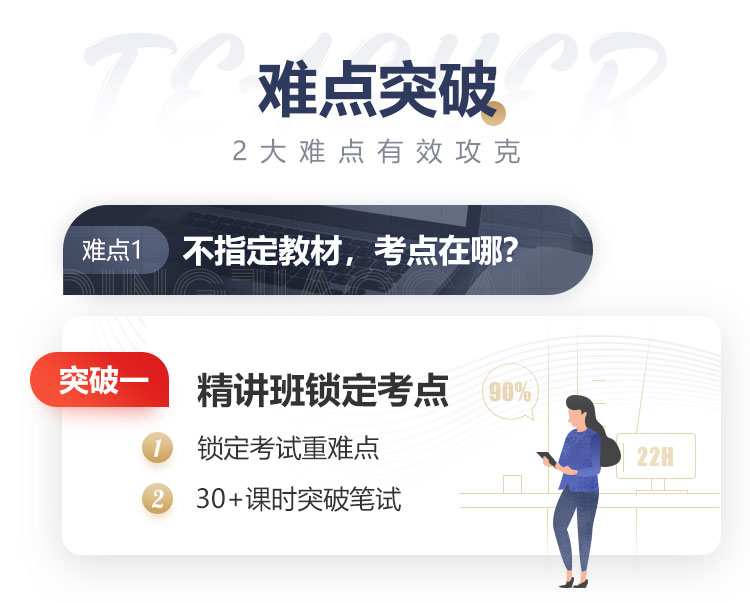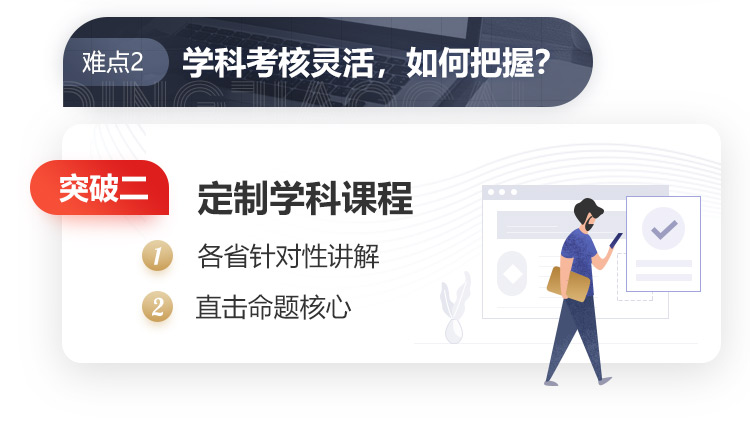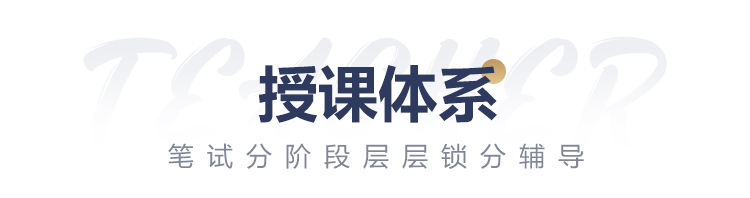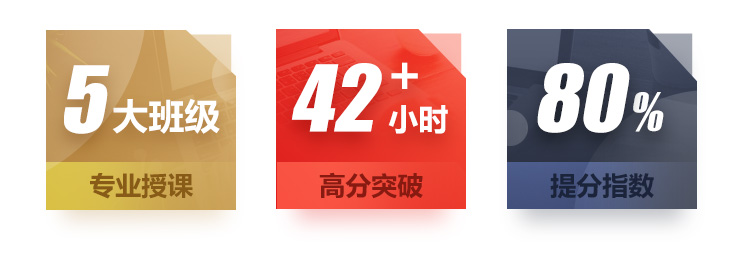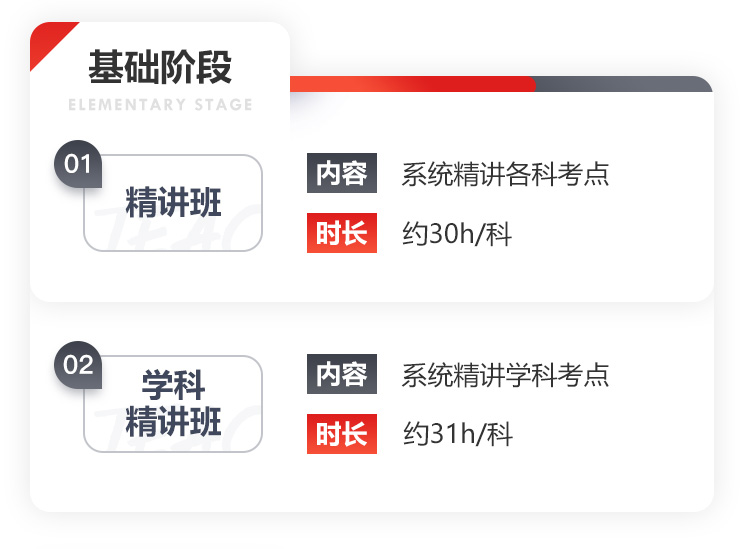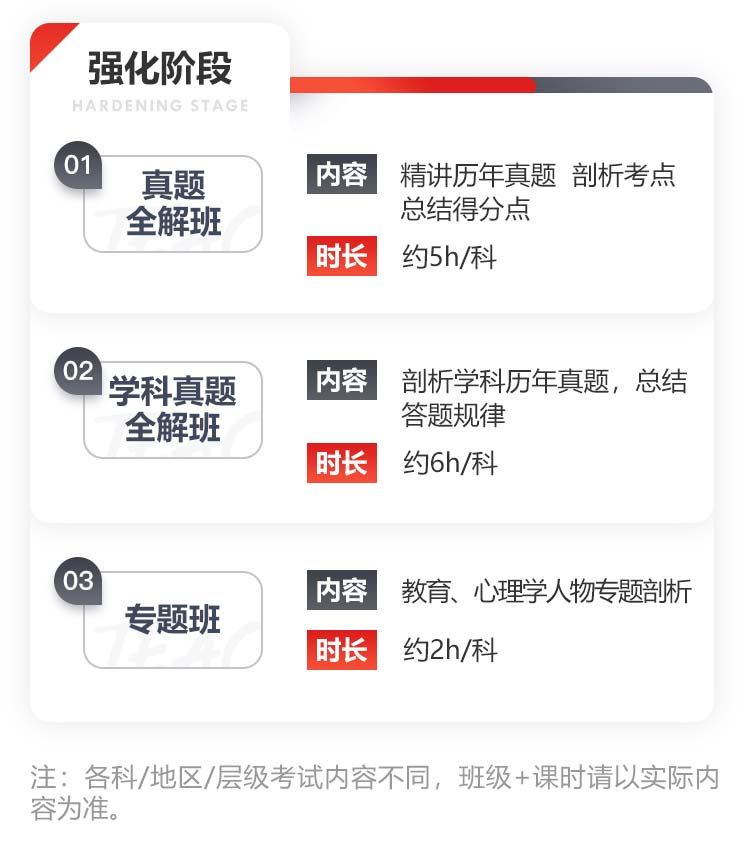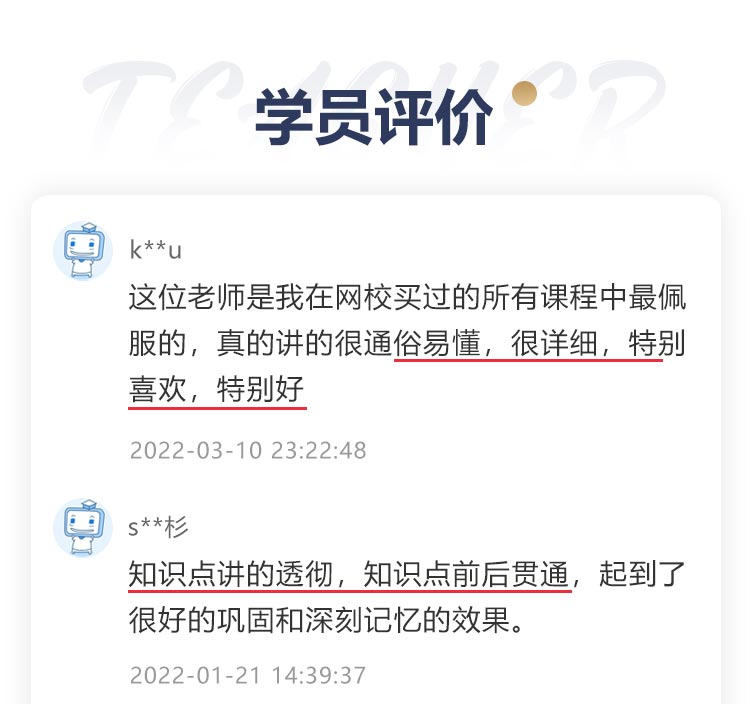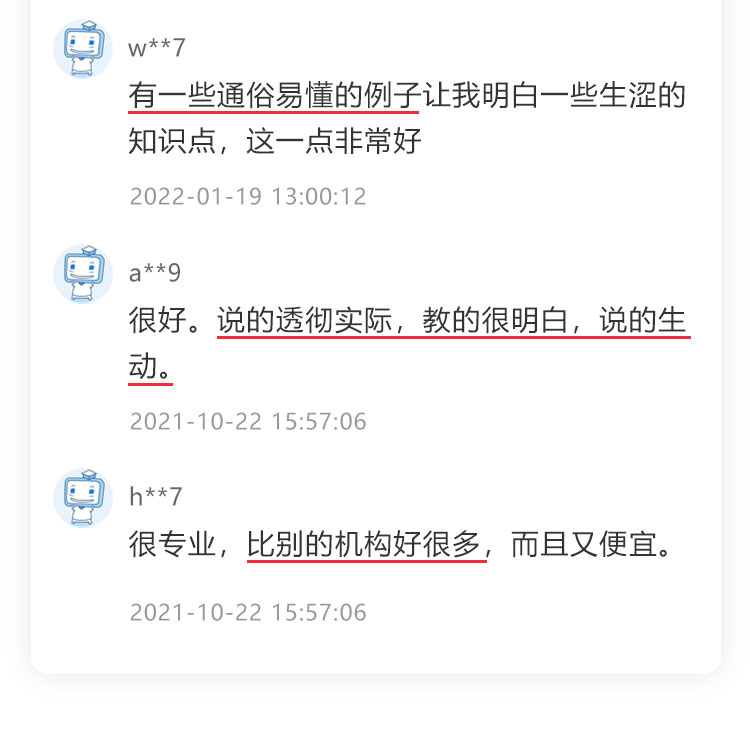### 2022教师招聘 笔试套餐班

• 全科笔试套餐班
• 单科笔试套餐班
• 两科笔试套餐班

• （统考版）中小学教育理论基础+公共基础+学科
• （非统考版）中小学教育理论基础+公共基础+学科
• （中小学）教育理论+公共基础+学科
• （中小学）教育理论+公共基础+学科
• （中小学/幼儿）公共基础知识
• （幼儿）教育理论基础
• （幼儿）教育理论基础
• （幼儿）教育理论基础
• （中小学）教育基础理论
• （中小学）教育基础理论
• 中学教育综合
• 中小学教育综合知识
• 小学教育综合知识
• 中小学教育公共基础
• 语文学科
• 语文学科
• 语文学科
• 语文学科
• 语文学科
• 语文学科
• 数学学科
• 数学学科
• 数学学科
• 数学学科
• 数学学科
• 数学学科
• 英语学科
• 英语学科
• 英语学科
• 英语学科
• 英语学科
• 英语学科
• （统考版）中小学教育理论基础
• （非统考版）中小学教育理论基础
• 中小学教育理论+学科
• 小学教育综合知识+学科
• 中学教育综合知识+学科
• 中小学教育公共基础+学科
• （统考版）中小学教育理论基础+公共基础
• （非统考版）中小学教育理论基础+公共基础
• 中小学教育综合知识+学科
• 中小学教育综合知识+学科
• 中小学教育理论+公共基础
• 中小学教育理论+公共基础
• 幼儿教育理论+公共基础
• （非统考版）中小学教育理论基础+学科
• （统考版）中小学教育理论基础+学科

• 新用户注册,即送6张优惠券,购课即可抵用
• 累计支付满300,再次购课享8.5折,新老优惠不同享